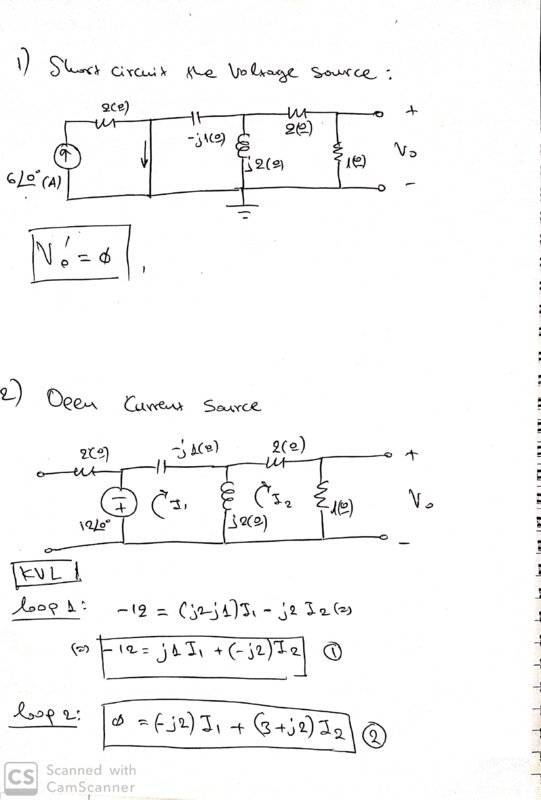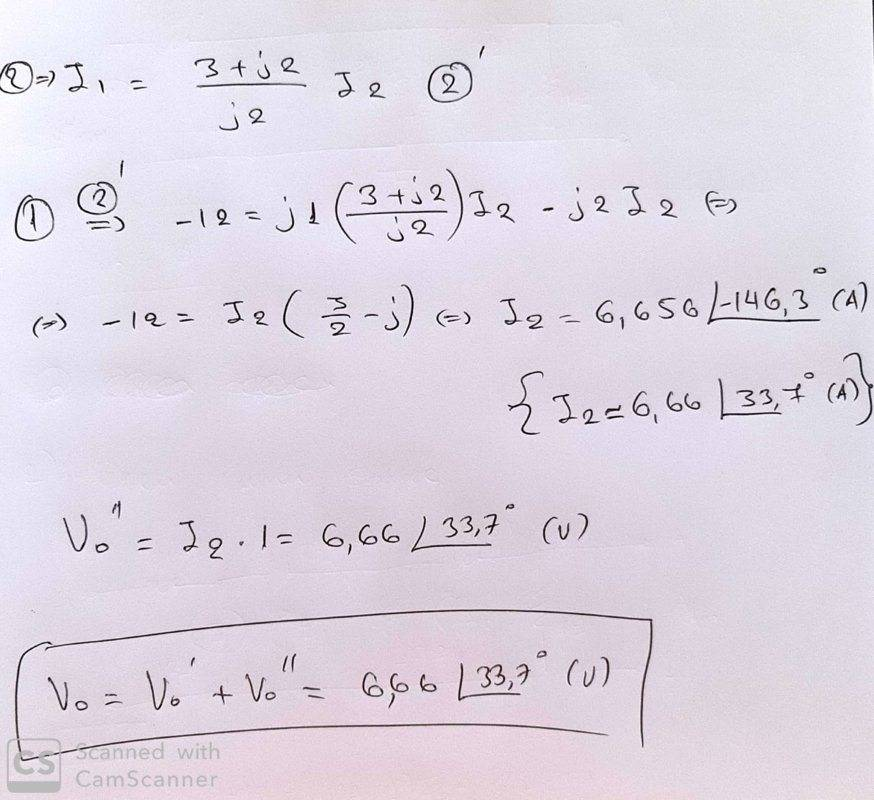# Find the Vo of the AC circuit

• Michael_0039

#### Michael_0039

This is the circuit: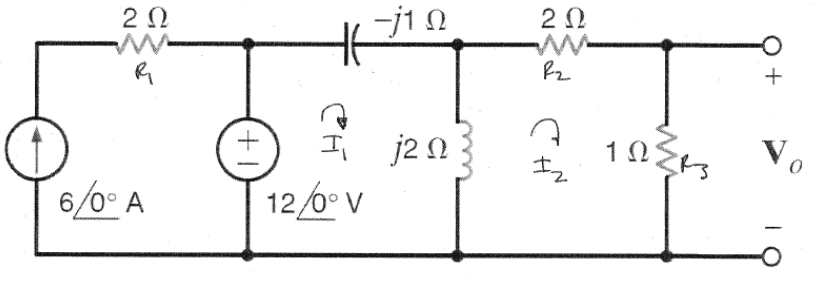I try with KVL: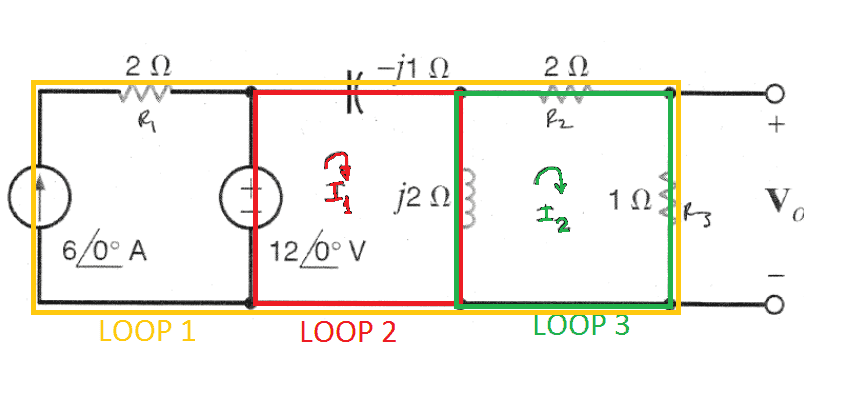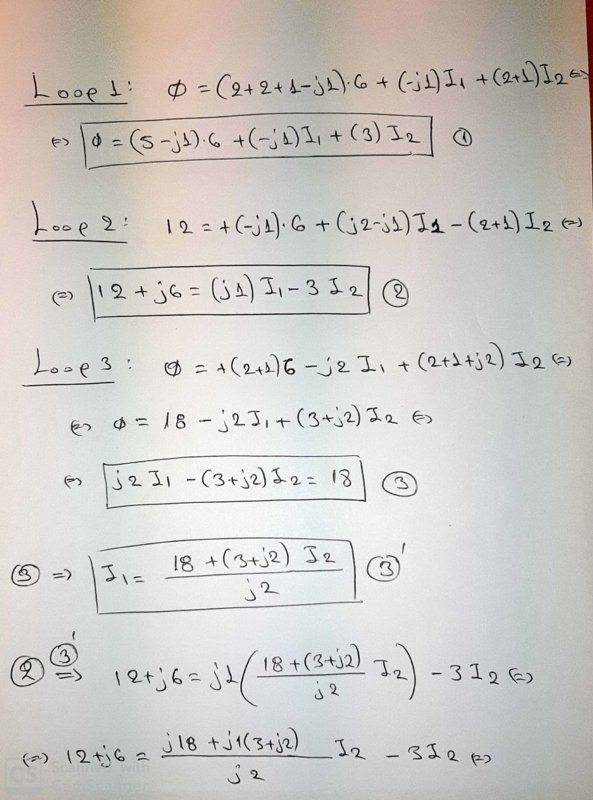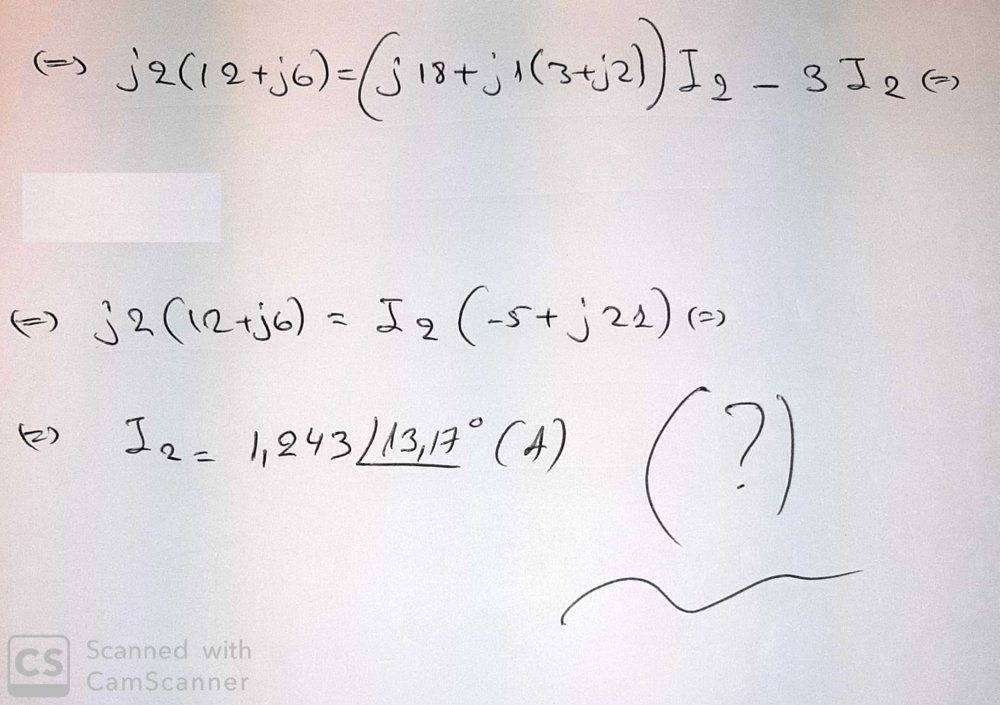But the given answer is 6.66L33.67 Volts. So, I'm confused, any suggestions ?

May I ask a question ?
I assume that you wrote a zero on the left side of the first loop equation, but why ?

The 6 amp current source injects 6 amps into the node at the top of the 12 volt voltage source, and the 2 ohm resistor doesn't change that fact. Injecting 6 amps into a voltage source doesn't change the voltage of the 12 volt source. The net result of what I've said so far is that you can get rid of the current source and the resistor in series with it; those things have no effect on Vo. Now you have only two meshes to solve.

•Michael_0039
May I ask a question ?
I assume that you wrote a zero on the left side of the first loop equation, but why ?
Because in this loop there is no Voltage source. The same on loop 3.